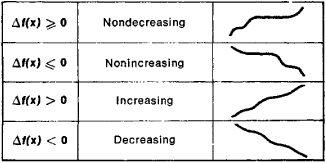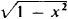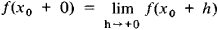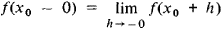# Monotonic Function

(redirected from Non-decreasing function)

## monotonic function

[¦män·ə¦tän·ik ′fəŋk·shən]
(mathematics)
McGraw-Hill Dictionary of Scientific & Technical Terms, 6E, Copyright © 2003 by The McGraw-Hill Companies, Inc.
The following article is from The Great Soviet Encyclopedia (1979). It might be outdated or ideologically biased.

## Monotonic Function

(or monotone function), a function whose increments Δf(x) = f(x′) − f(x) do not change sign when Δx = x′ − x > 0; that is, the increments are either always nonnegative or always nonpositive. Somewhat inaccurately, a monotonic function can be defined as a function that always varies in the same direction. Different types of monotonic functions are represented in Figure 1. For example, the function y = x3 is an increasing function. If a function f(x) has a derivative f′(x) that is nonnegative at every point and that vanishes only at a finite number of individual points, then f(x) is an increasing function. Similarly, if f′(x) ≤ 0 and vanishes only at a finite number of points, then f(x) is a decreasing function.Figure 1

A monotonicity condition can hold either for all x or for x on a given interval. In the latter case, the function is said to be monotonic on this interval. For example, the function y =increases on the interval [−1,0] and decreases on the interval [0, +1]. A monotonic function is one of the simplest classes of functions and is continually encountered in mathematical analysis and the theory of functions. If f(x) is a monotonic function, then the following limits exist for any X0:andReferences in periodicals archive ?
Since [[not upsilon].sub.[GAMMA]] is a non-decreasing function, we have
(a3) R(t, *) is a left continuous non-decreasing function for any t, R(*, s) is locally integrable for any s, and R(t, h(t)) = 0, R(t, [t.sup.+]) = 1 for any t [greater than or equal to] 0;
Moreover, since ([phi],[psi]) [member of] [PHI] x [PHI] [subset.bar] [PHI] x [PHI], then [phi] and [psi] are non-decreasing functions, which yields [T.sub.max]([phi],[psi]) is also a non-decreasing function.
[16,24] For all x, y [member of] X, (X, M, x) is non-decreasing function.
If (|[H.sub.m](e*)|/|[H.sub.m+1](e*)|) forms a non-decreasing function of m then ([[alpha].sub.m] = [[alpha].sub.m+1]) also form a non-decreasing function of m, where [[alpha].sub.m] = [square root of [d.sub.m]]|[H.sub.m](e*)|.
The function should be continuous, differentiable and monotonically non-decreasing function. The activation function used in this paper is Bipolar Sigmoid Function,
with [mu]{t) a non-decreasing function such that [[integral].sup.[infinity].sub.1] 1/[t.sup.k] d[mu](t) < [integral] and k [member of] N.
It is well known that any nonlinear, smooth, differentiable, and preferably non-decreasing function can be used as AF in hidden layer.
From Theorem 1, for n + 1 [greater than or equal to] 4 log q, we note that the function [theta](n, q) is non-decreasing function of n, it follows that the function D(n, q) is decreasing function of [theta].
The "constants" in those combinations will be functions cp(t) where c is any positive real number and p(t) is any positive continuous non-decreasing function. This combination will be mentioned in 3., 4., and 5.
a) f([x]) > 0; b) f([x]) is a non-decreasing function; c) for each n > 0; [phi] (n) = lim [x] [right arrow] [infinity] f(n[x]/f([x])
X is said to be smaller than Y in simple stochastic ordering, denoted by X [[less than or equal to].sub.st] Y, if G(t) [less than or equal to] F(t) for all t [greater than or equal to] 0, or, equivalently, if E([phi](X)) [less than or equal to] E([phi](Y)) for all non-decreasing functions [phi] for which the expectations exist.

Site: Follow: Share:
Open / Close